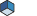Open Inventor Release 2023.2.1
Searching...
No Matches
SbVec4s Class Reference4D vector class. More...

`#include <Inventor/SbVec.h>`

## Public Member Functions

SbVec4s ()
Default constructor.

SbVec4s (const short v)
Constructor given 4 components.

SbVec4s (short x, short y, short z, short w)
Constructor given 4 components.

int32_t dot (const SbVec4s &v) const
Returns dot (inner) product of vector and another vector.

const short * getValue () const
Returns vector components.

void getValue (short &x, short &y, short &z, short &w) const
Returns vector components.

void negate ()
Negates each component of vector in place.

SbVec4ssetValue (const short v)
Sets vector components.

SbVec4ssetValue (short x, short y, short z, short w)
Sets vector components.

short & operator[] (int i)
Accesses indexed component of vector.

const short & operator[] (int i) const

SbVec4soperator*= (int d)
Component-wise scalar multiplication operator.

SbVec4soperator*= (double d)
Component-wise scalar multiplication operator.

SbVec4soperator/= (int d)
Component-wise scalar division operator.

SbVec4soperator/= (double d)
Component-wise scalar division operator.

SbVec4soperator+= (const SbVec4s &u)

SbVec4soperator-= (const SbVec4s &u)
Component-wise vector subtraction operator.

SbVec4s operator- () const
Nondestructive unary negation - returns a new vector.

template<typename T >
SbVec4s (const T &v)
Constructor that converts an arbitrary SbVec4 to an SbVec4s.

## Friends

SbVec4s operator* (const SbVec4s &v, int d)
Component-wise binary scalar multiplication operator.

SbVec4s operator* (const SbVec4s &v, double d)
Component-wise binary scalar multiplication operator.

SbVec4s operator* (int d, const SbVec4s &v)
Component-wise binary scalar multiplication operator.

SbVec4s operator* (double d, const SbVec4s &v)
Component-wise binary scalar multiplication operator.

SbVec4s operator/ (const SbVec4s &v, int d)
Component-wise binary scalar division operator.

SbVec4s operator/ (const SbVec4s &v, double d)
Component-wise binary scalar division operator.

SbVec4s operator+ (const SbVec4s &v1, const SbVec4s &v2)

SbVec4s operator- (const SbVec4s &v1, const SbVec4s &v2)
Component-wise binary vector subtraction operator.

int operator== (const SbVec4s &v1, const SbVec4s &v2)
Equality comparison operator.

int operator!= (const SbVec4s &v1, const SbVec4s &v2)
Inequality comparison operator.

std::ostream & operator<< (std::ostream &os, const SbVec4s &v)
Writes the vector to the specified output stream.

## Detailed Description4D vector class.

4D vector class used to store 4D integer vectors and points. This class is used throughout Open Inventor for arguments and return values.

Definition at line 2824 of file SbVec.h.

## ◆ SbVec4s() [1/4]

 SbVec4s::SbVec4s ( )
inline

Default constructor.

The vector is initialized with zeros.

Definition at line 2830 of file SbVec.h.

## ◆ SbVec4s() [2/4]

 SbVec4s::SbVec4s ( const short v )
inlineexplicit

Constructor given 4 components.

Definition at line 2835 of file SbVec.h.

## ◆ SbVec4s() [3/4]

 SbVec4s::SbVec4s ( short x, short y, short z, short w )
inline

Constructor given 4 components.

Definition at line 2840 of file SbVec.h.

## ◆ SbVec4s() [4/4]

template<typename T >
 SbVec4s::SbVec4s ( const T & v )
inlineexplicit

Constructor that converts an arbitrary SbVec4 to an SbVec4s.

Definition at line 2971 of file SbVec.h.

## ◆ dot()

 int32_t SbVec4s::dot ( const SbVec4s & v ) const
inline

Returns dot (inner) product of vector and another vector.

Definition at line 2845 of file SbVec.h.

## ◆ getValue() [1/2]

 const short * SbVec4s::getValue ( ) const
inline

Returns vector components.

Definition at line 2851 of file SbVec.h.

## ◆ getValue() [2/2]

 void SbVec4s::getValue ( short & x, short & y, short & z, short & w ) const

Returns vector components.

## ◆ negate()

 void SbVec4s::negate ( )

Negates each component of vector in place.

## ◆ operator*=() [1/2]

 SbVec4s & SbVec4s::operator*= ( double d )

Component-wise scalar multiplication operator.

## ◆ operator*=() [2/2]

 SbVec4s & SbVec4s::operator*= ( int d )

Component-wise scalar multiplication operator.

## ◆ operator+=()

 SbVec4s & SbVec4s::operator+= ( const SbVec4s & u )

## ◆ operator-()

 SbVec4s SbVec4s::operator- ( ) const

Nondestructive unary negation - returns a new vector.

## ◆ operator-=()

 SbVec4s & SbVec4s::operator-= ( const SbVec4s & u )

Component-wise vector subtraction operator.

## ◆ operator/=() [1/2]

 SbVec4s & SbVec4s::operator/= ( double d )
inline

Component-wise scalar division operator.

Definition at line 2897 of file SbVec.h.

## ◆ operator/=() [2/2]

 SbVec4s & SbVec4s::operator/= ( int d )

Component-wise scalar division operator.

## ◆ operator[]() [1/2]

 short & SbVec4s::operator[] ( int i )
inline

Accesses indexed component of vector.

Definition at line 2877 of file SbVec.h.

## ◆ operator[]() [2/2]

 const short & SbVec4s::operator[] ( int i ) const
inline

Definition at line 2878 of file SbVec.h.

## ◆ setValue() [1/2]

 SbVec4s & SbVec4s::setValue ( const short v )

Sets vector components.

## ◆ setValue() [2/2]

 SbVec4s & SbVec4s::setValue ( short x, short y, short z, short w )

Sets vector components.

## ◆ operator!=

 int operator!= ( const SbVec4s & v1, const SbVec4s & v2 )
friend

Inequality comparison operator.

Definition at line 2959 of file SbVec.h.

## ◆ operator* [1/4]

 SbVec4s operator* ( const SbVec4s & v, double d )
friend

Component-wise binary scalar multiplication operator.

## ◆ operator* [2/4]

 SbVec4s operator* ( const SbVec4s & v, int d )
friend

Component-wise binary scalar multiplication operator.

## ◆ operator* [3/4]

 SbVec4s operator* ( double d, const SbVec4s & v )
friend

Component-wise binary scalar multiplication operator.

Definition at line 2930 of file SbVec.h.

## ◆ operator* [4/4]

 SbVec4s operator* ( int d, const SbVec4s & v )
friend

Component-wise binary scalar multiplication operator.

Definition at line 2925 of file SbVec.h.

## ◆ operator+

 SbVec4s operator+ ( const SbVec4s & v1, const SbVec4s & v2 )
friend

## ◆ operator-

 SbVec4s operator- ( const SbVec4s & v1, const SbVec4s & v2 )
friend

Component-wise binary vector subtraction operator.

## ◆ operator/ [1/2]

 SbVec4s operator/ ( const SbVec4s & v, double d )
friend

Component-wise binary scalar division operator.

Definition at line 2939 of file SbVec.h.

## ◆ operator/ [2/2]

 SbVec4s operator/ ( const SbVec4s & v, int d )
friend

Component-wise binary scalar division operator.

## ◆ operator<<

 std::ostream & operator<< ( std::ostream & os, const SbVec4s & v )
friend

Writes the vector to the specified output stream.

Definition at line 3693 of file SbVec.h.

## ◆ operator==

 int operator== ( const SbVec4s & v1, const SbVec4s & v2 )
friend

Equality comparison operator.

The documentation for this class was generated from the following file: